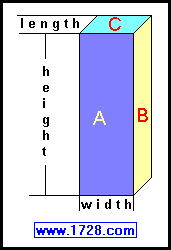Rectangular Prism (Solid) Area and Volume Calculator Scroll down for instructions and definitions If you need a Triangular Prism Calculator, click here.

This calculator is extremely easy to use. Input the 3 dimensions (length, width and height), click on "CALCULATE" and you'll have your answers.

Rectangular Prisms (or Solids)The above figure is called a rectangular prism.
This is a geometric solid having 6 faces all of which are rectangles and each pair of opposite faces are congruent.
Volume = length × width × height
Area of Face 'A' = height × width
Area of Face 'B' = height × length
Area of Face 'C' = width × length

Rectangular prisms you might encounter in everyday life are cereal boxes, shoe boxes and wooden planks.

Significant Figures >>>
The default setting is for 5 significant figures but you can change that by inputting another number in the box above.

Answers are displayed in scientific notation and for easier readability, numbers between .001 and 1,000 will be displayed in standard format (with the same number of significant figures.)
The answers should display properly but there are a few browsers that will show no output whatsoever. If so, enter a zero in the box above. This eliminates all formatting but it is better than seeing no output at all.